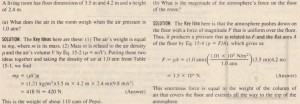# Density and Pressure

Density and Pressure

When we discuss rigid bodies, we are concerned with particular lumps of matter, such as wooden blocks, baseballs, or metal rods. Physical quantities that we find useful, and in whose terms we express Newton’s laws, are I1.7aSS and force. We might speak, for example, of a 3.6 kg block acted on by a 25 N force. With fluids, we are more interested in the extended substance. and in properties that can vary from point to point in that substance. It. is more useful to speak of
density and pressure than of mass and force.

Density

To find the density p of a fluid at any point, we isolate a small volume element AV around that point and measure the mass Am of the fluid contained within that element. The density is then.In theory, the density at any point in a fluid is the limit of this ratio as the volume  element AV at that point is made smaller and smaller. In practice. we assume that fluid sample is large compared to atomic dimensions and thus is smooth” (with uniform density), rather than “lumpy” with atoms. This assumption allows us towhere m and V are the mass and volume of the sample. Density is a scalar property; its SI unit is the kilogram per cubic meter. Table IS-1 shows the densities of some substances and the average densities of some objects. Note that the density of a gas (see Air in the table) varies considerably with pressure, but the density of a liquid (see Water) does not: that is. gases are readily compressible but liquids are not.In theory, the pressure at any point in the fluid is the limit of this ratio as the area of the piston. centered on that point, is made smaller and smaller. However, if the force is uniform over a flat area A, we can write Eq. IS-3 asSample Problem